# Measurement Lesson #4 Surface Area of Pyramids & Cones

 Math 10C Measurement Lesson #4 Surface Area of Pyramids & Cones Objective: By the end of this lesson you will be able to: Recall: To find surface area of an object with flat faces: Surface are is measured in _____________ units. A right pyramid is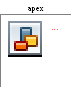In the diagram: b = h = s = The area of a triangle is: To find the area of a triangular face, you must use the _______________________ as the height of each triangular face. e.g. 1) Find the area of the pyramid above (including the base), if b = 15”, h = 17.5”, and s = 19”. T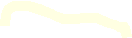he area of the triangular faces is also known as the ________________ ________ (denoted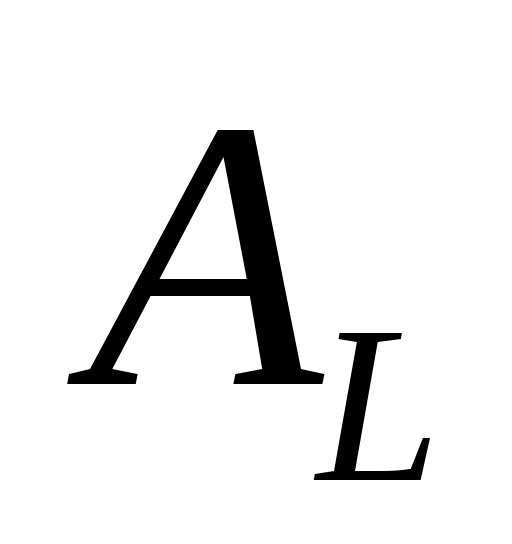). A regular tetrahedron is e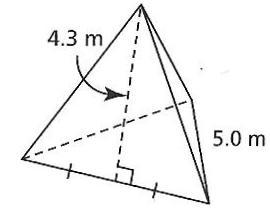.g. 2) Find the surface area of the regular tetrahedron shown. Sometimes the height of the pyramid is given instead of the slant height. Then we have to use the ________________________ _____________ to find the slant height. e.g. 3) A skylight has the shape of a right pyramid. The square base measures 1.2 m by 1.2 m, and the height is 1.6 m. a) Draw a sketch of this pyramid.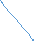Find the slant height of the pyramid to the nearest tenth.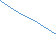Determine the area of glass that would be needed for this skylight to the nearest tenth. (Hint: there is no glass needed for the base of the pyramid.) There is another formula for the surface area of a right pyramid: Surface Area of a Right Pyramid with a Regular Polygon Base: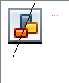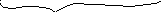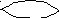e.g. 4) A paperweight is in the shape of a hexagonal pyramid. It has a base side length of 2.8 cm and a slant height of 7.8 cm. The area of the base is 20.4 cm2. Determine the surface area of the paperweight using the formula above.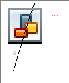Share with your friends: